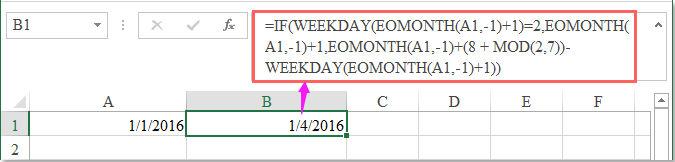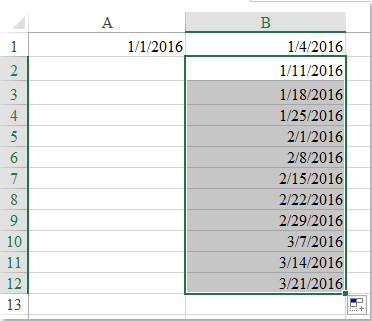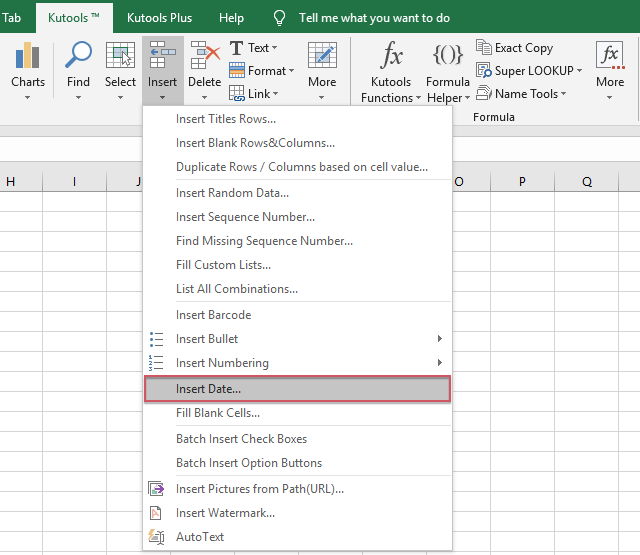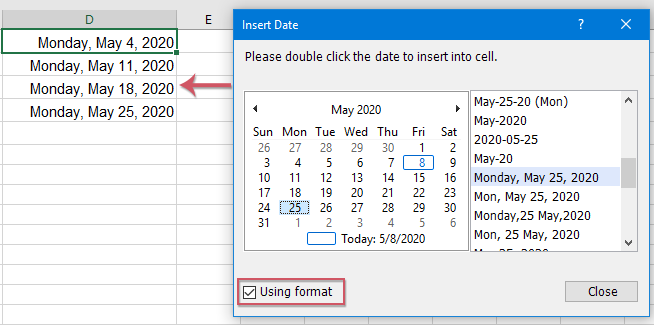Note: The other languages of the website are Google-translated. Back to English

## 如何在Excel中列出一个月中的所有星期一/星期五？

#### 用公式列出一个月或一年中的所有星期一/星期五

1. 请在单元格中输入要列出的每个月的每个星期一或星期五的日期，例如，我将在单元格A1中输入1/2016/1。

2. 然后输入以下公式： =IF(WEEKDAY(EOMONTH(A1,-1)+1)=2,EOMONTH(A1,-1)+1,EOMONTH(A1,-1)+(8 + MOD(2,7))-WEEKDAY(EOMONTH(A1,-1)+1)) 进入单元格B1，然后按 输入 获取每月第一个星期一的密钥。 看截图：=IF(WEEKDAY(EOMONTH(A1,-1)+1)=3,EOMONTH(A1,-1)+1,EOMONTH(A1,-1)+(8 + MOD(3,7))-WEEKDAY(EOMONTH(A1,-1)+1))

=IF(WEEKDAY(EOMONTH(A1,-1)+1)=4,EOMONTH(A1,-1)+1,EOMONTH(A1,-1)+(8 + MOD(4,7))-WEEKDAY(EOMONTH(A1,-1)+1))

=IF(WEEKDAY(EOMONTH(A1,-1)+1)=5,EOMONTH(A1,-1)+1,EOMONTH(A1,-1)+(8 + MOD(5,7))-WEEKDAY(EOMONTH(A1,-1)+1))

=IF(WEEKDAY(EOMONTH(A1,-1)+1)=6,EOMONTH(A1,-1)+1,EOMONTH(A1,-1)+(8 + MOD(6,7))-WEEKDAY(EOMONTH(A1,-1)+1))

=IF(WEEKDAY(EOMONTH(A1,-1)+1)=7,EOMONTH(A1,-1)+1,EOMONTH(A1,-1)+(8 + MOD(7,7))-WEEKDAY(EOMONTH(A1,-1)+1))

=IF(WEEKDAY(EOMONTH(A1,-1)+1)=1,EOMONTH(A1,-1)+1,EOMONTH(A1,-1)+(8 + MOD(1,7))-WEEKDAY(EOMONTH(A1,-1)+1))

3. 显示第一个星期一后，请继续输入以下公式： = B1 + 7 要获取第二个星期一，然后向下拖动填充手柄以根据需要列出一个月或一年中的所有星期一，请参见屏幕截图：#### 使用Kutools for Excel列出一个月或一年中的所有星期一/星期五

 : 带有300多个便捷的Excel加载项，可以在30天内免费试用.

1。 选择一个单元格以插入日期，然后单击 库工具 > 插页 > 插入日期，请参见屏幕截图：2。 在 插入日期 对话框中，显示一个月日历，您可以双击日期以将其插入到单元格中，也可以通过选中以下日期来更改日期格式 使用格式 选项以激活日期格式列表框。 看截图：#### 演示：使用Kutools for Excel列出一个月或一年中的所有星期一/星期五

Kutools for Excel：具有300多个方便的Excel加载项，可以在30天内免费试用，没有任何限制。

### 最佳办公效率工具

#### Kutools for Excel解决了您的大多数问题，并使您的生产率提高了80％

• 重用: 快速插入 复杂的公式，图表 以及您以前使用过的任何东西； 加密单元 带密码 创建邮件列表 并发送电子邮件...
• 超级公式栏 （轻松编辑多行文本和公式）； 阅读版式 （轻松读取和编辑大量单元格）； 粘贴到过滤范围...
• 合并单元格/行/列 不会丢失数据； 拆分单元格内容； 合并重复的行/列...防止细胞重复； 比较范围...
• 选择重复或唯一 行; 选择空白行 （所有单元格都是空的）； 超级查找和模糊查找 在许多工作簿中； 随机选择...
• 确切的副本 多个单元格，无需更改公式参考； 自动创建参考 到多张纸； 插入项目符号，复选框等...
• 提取文字，添加文本，按位置删除， 删除空间; 创建和打印分页小计； 在单元格内容和注释之间转换...
• 超级滤镜 （将过滤方案保存并应用于其他工作表）； 高级排序 按月/周/日，频率及更多； 特殊过滤器 用粗体，斜体...
• 结合工作簿和工作表; 根据关键列合并表； 将数据分割成多个工作表; 批量转换xls，xlsx和PDF...
• 超过300种强大功能. 支持 Office / Excel 2007-2021 和 365。支持所有语言。 在您的企业或组织中轻松部署。 完整功能 30 天免费试用。 60 天退款保证。#### Office选项卡为Office带来了选项卡式界面，使您的工作更加轻松

• 在Word，Excel，PowerPoint中启用选项卡式编辑和阅读，发布者，Access，Visio和Project。
• 在同一窗口的新选项卡中而不是在新窗口中打开并创建多个文档。
• 每天将您的工作效率提高50％，并减少数百次鼠标单击！It worked for me.. fo rFridays A1= 06/01/2017 B1= 13/01/2017= IF(WEEKDAY(EOMONTH(A1,-1)+1)=6,EOMONTH(A1,-1)+1,EOMONTH(A1,-1)+(8 + MOD(6,7))-WEEKDAY(EOMONTH(A1,-1)+1)) 20/01/2017 = IF(WEEKDAY(EOMONTH(B1,-1)+1)=6,EOMONTH(B1,-1)+1,EOMONTH(B1,-1)+(8 + MOD(6,7))-WEEKDAY(EOMONTH(B1,-1)+1))+7 27/01/2017=IF(WEEKDAY(EOMONTH(C1,-1)+1)=6,EOMONTH(C1,-1)+1,EOMONTH(C1,-1)+(8 + MOD(6,7))-WEEKDAY(EOMONTH(C1,-1)+1))+

2019 年也是：（第一个星期三不是 09 月 XNUMX 日吗？）

=IF(WEEKDAY(EOMONTH(A2,-1)+1)=1,EOMONTH(A2,-1)+2,IF(WEEKDAY(EOMONTH(A2,-1)+1)=2,EOMONTH(A2,-1)+1,EOMONTH(A2,-1)+(8+MOD(2,7))-WEEKDAY(EOMONTH(A2,-1)+1)))

=A1+MOD(8-工作日(A1,2),7)

0   产品特性

## 关注我们

Microsoft和Office徽标是Microsoft Corporation在美国和/或其他国家的商标或注册商标。